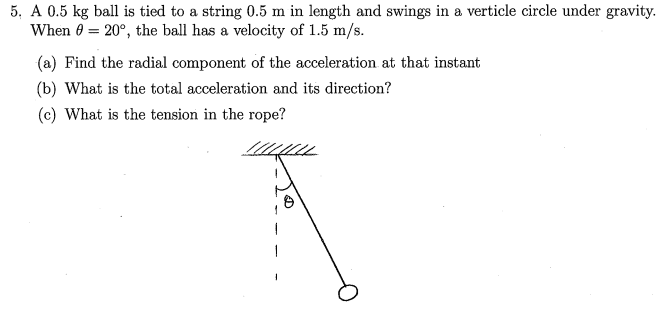# Rope being spun with mass

Panphobia

## Homework Statementmv^2/r
v^2/r

## The Attempt at a Solution

My physics midterm is tomorrow and I was doing review today, so I did this question, then looked at the professor's solutions. For the radius in both equations he used the length of the rope, but shouldn't the radius be Lsin20??? Also the way he positioned his y and x axis was that the Y axis was parallel to tension(EXTRA INFO).

Homework Helper
Hi Panphobia!(your professor can't spell "vertical"!)
For the radius in both equations he used the length of the rope, but shouldn't the radius be Lsin20??? Also the way he positioned his y and x axis was that the Y axis was parallel to tension(EXTRA INFO).

I think you're misunderstanding "swings in a vertical circle".

It means that the circle is in a vertical plane.(Lsin20° would be for a horizontal circle)

Panphobia
Yea that is what I meant, it is pointing down. So is my professor wrong? OHHHH CRAPP didn't know it was a pendulum, sorry man this question was dumb :(

Homework Helper
no, the ball is moving in the plane of the paper, and so the radius of the circle is LPanphobia
Yea I pointed out earlier that it is moving as a pendulum and not in a circle.

Homework Helper
if the radius of the circle is L, why would you use Lsin20° ?Panphobia
I figured that out earlier, I thought original that it the mass was spinning about the vertical axis at angle theta, THEN the radius would be from the end of the mass to the vertical axis. But later I read the question more in depth and found that it was a pendulum, and if it is a pendulum then the radius is the length of the rope.

(isn't that what i said?)
so is it all ok now?•